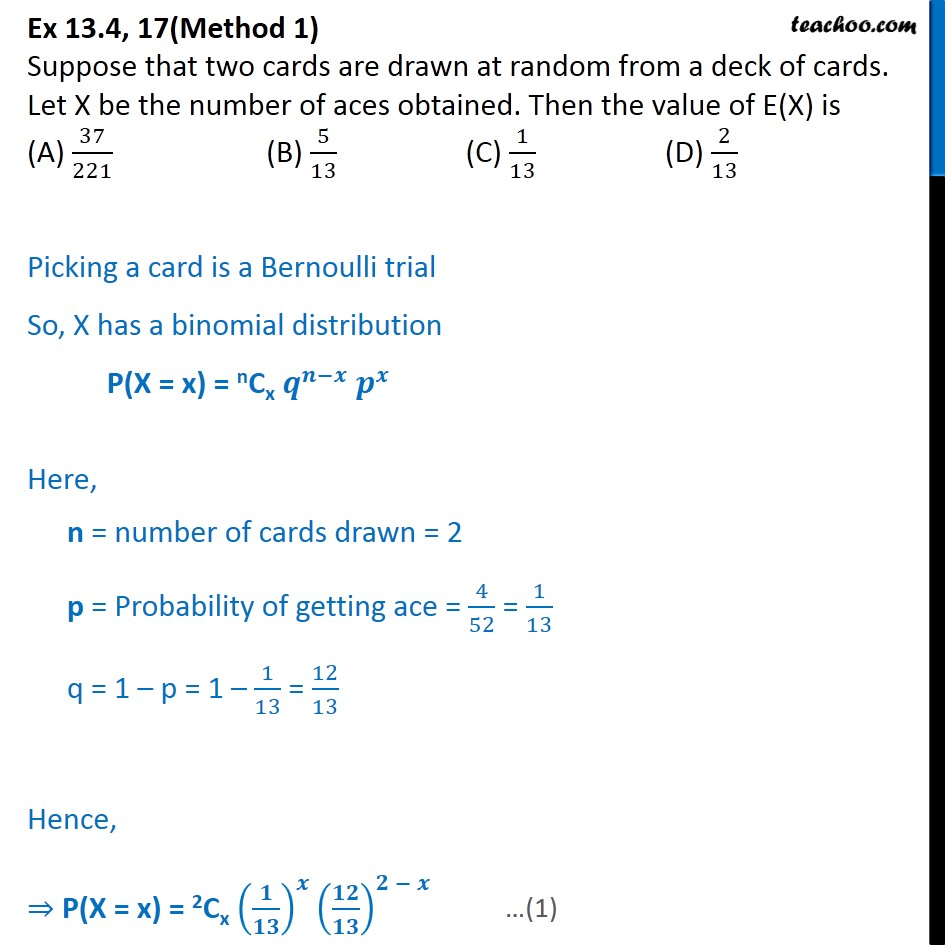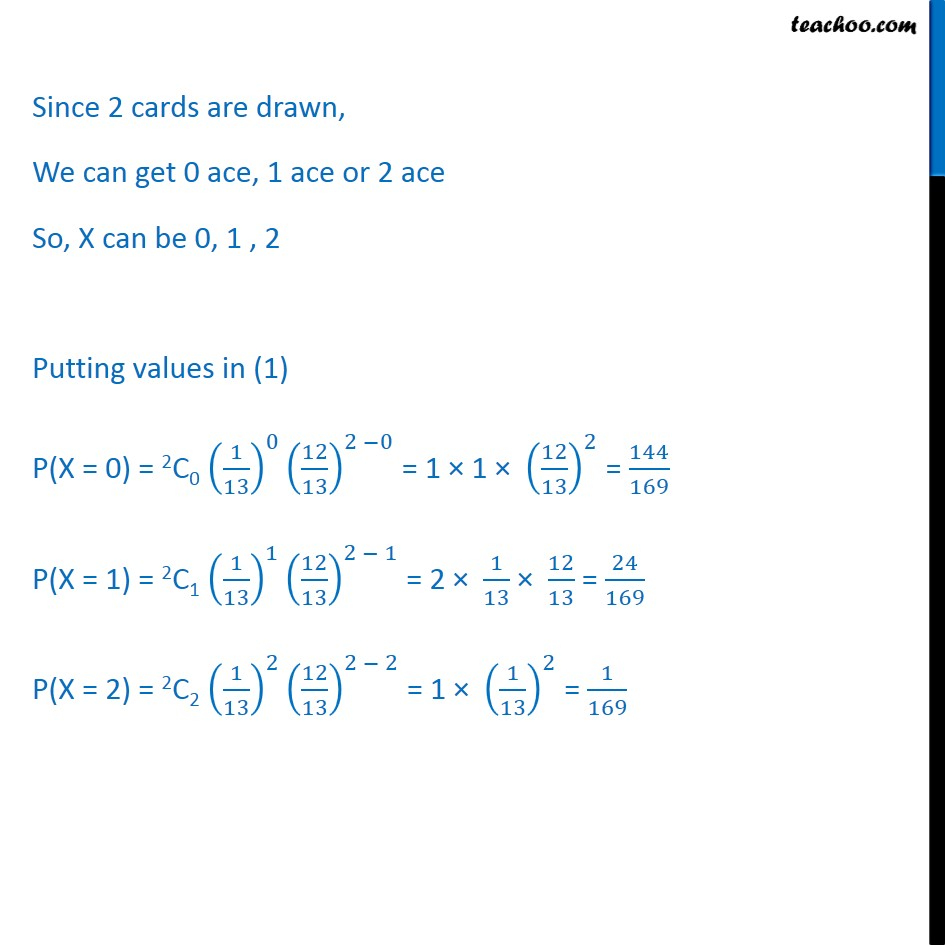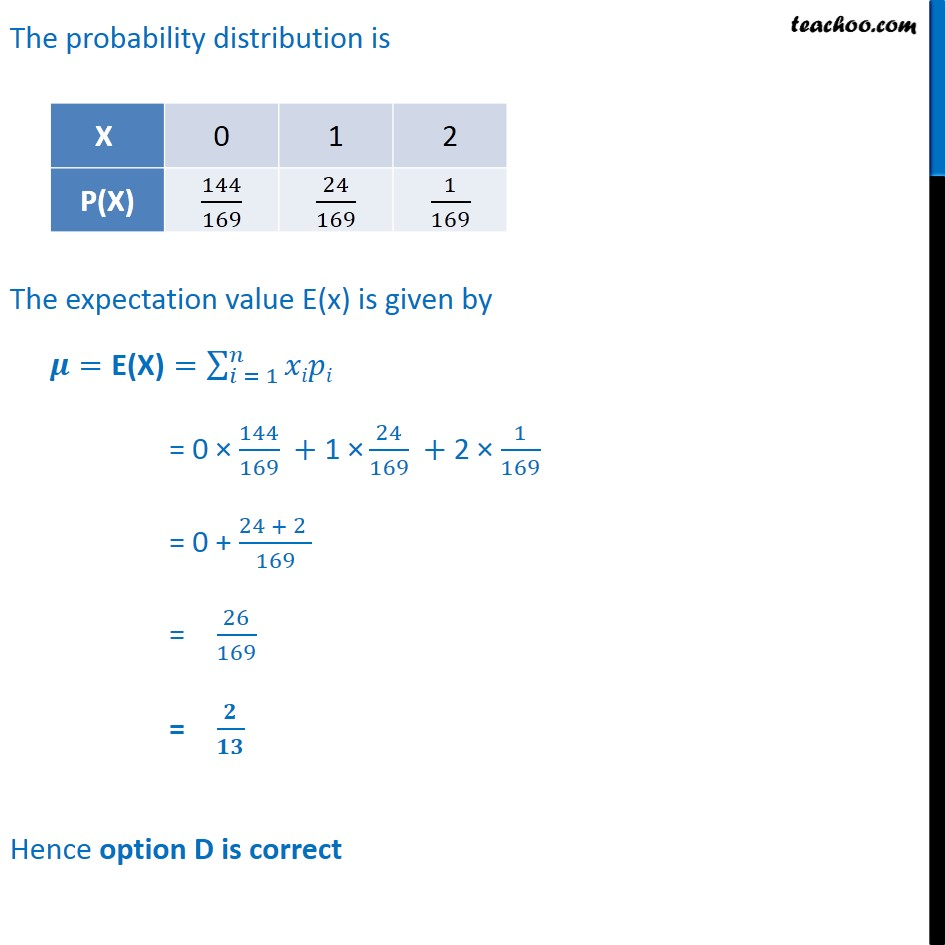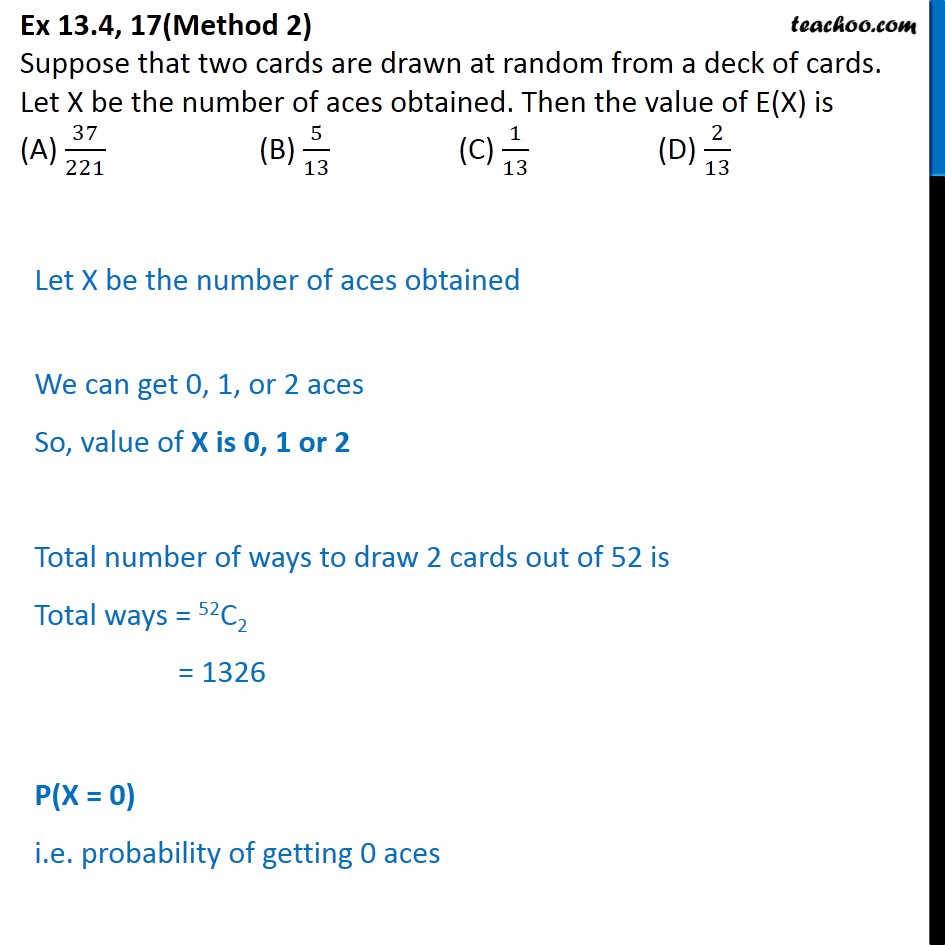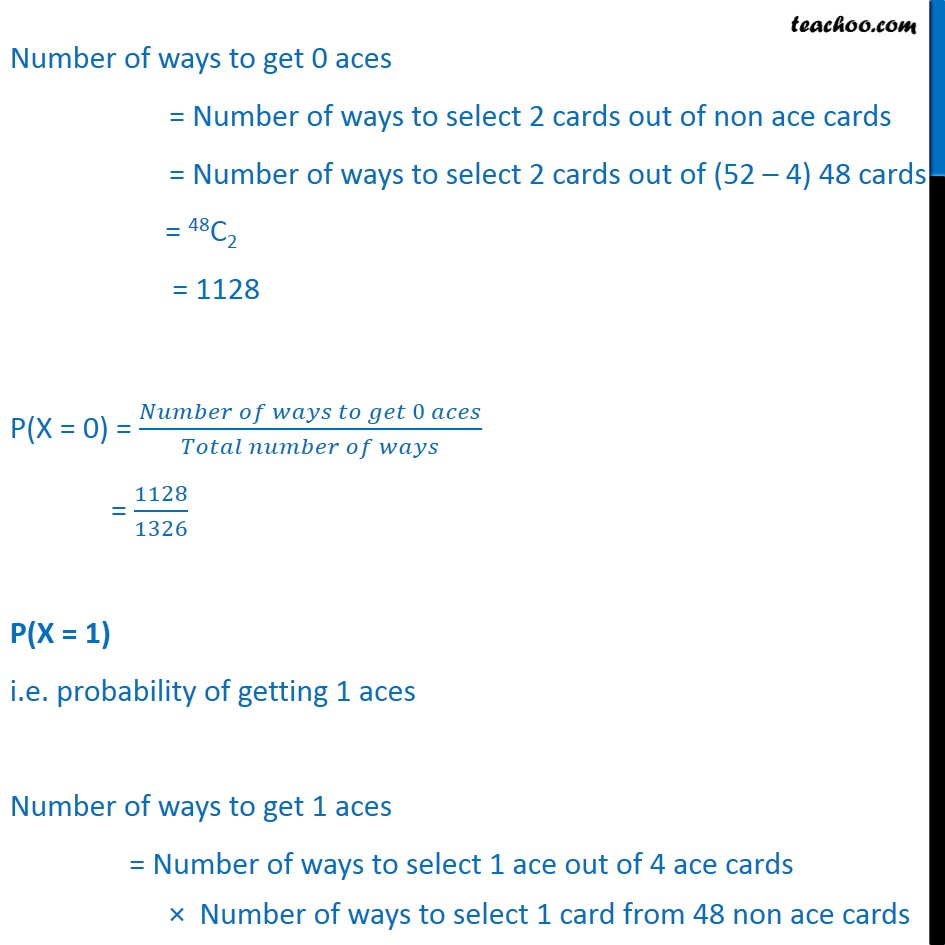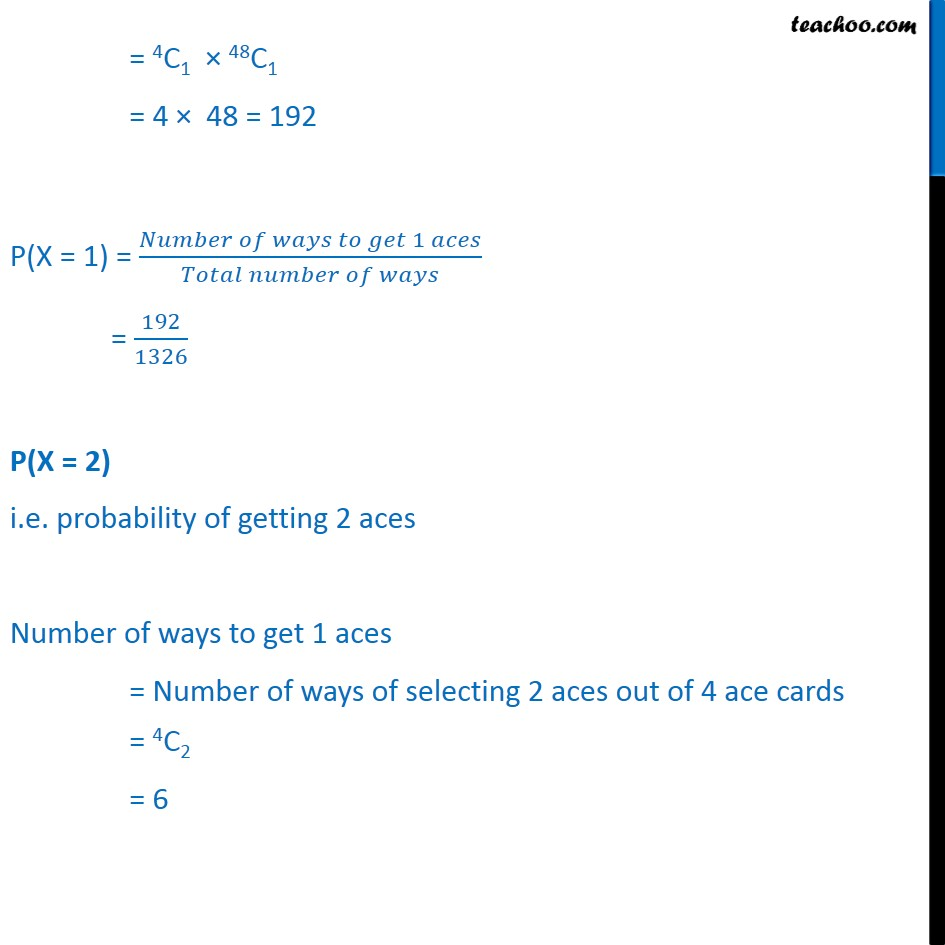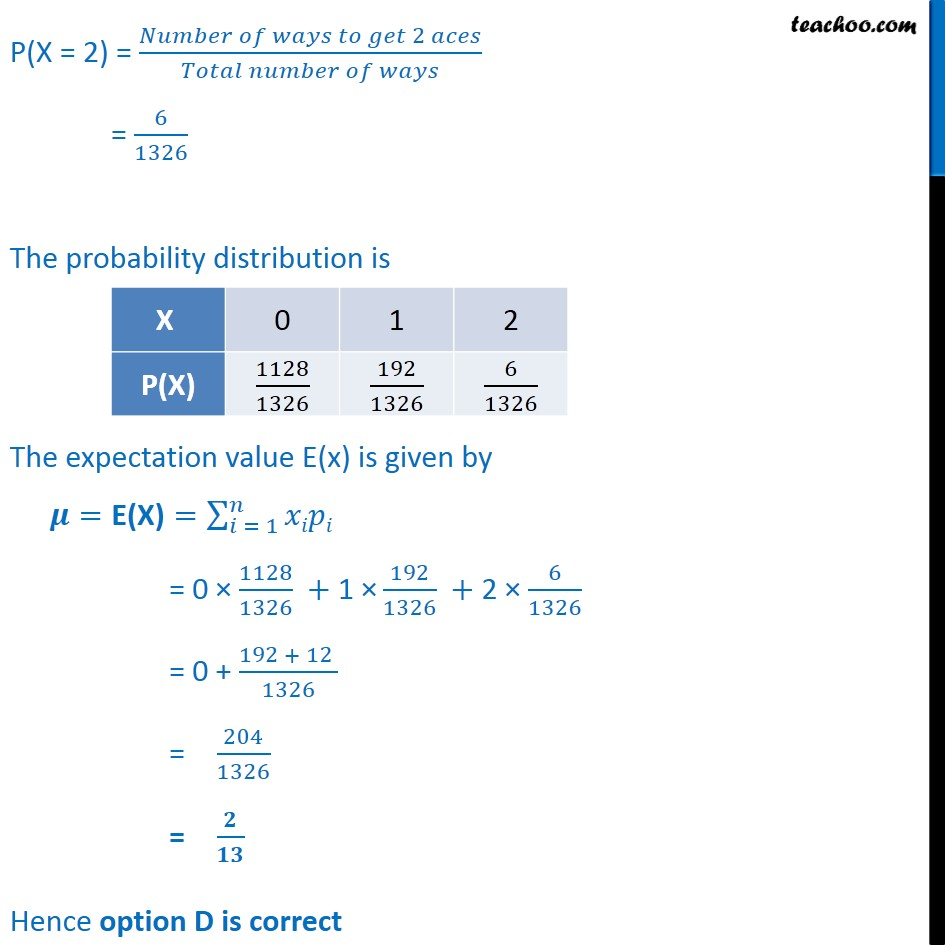1. Chapter 13 Class 12 Probability
2. Serial order wise
3. Ex 13.4

Transcript

Ex 13.4, 17(Method 1) Suppose that two cards are drawn at random from a deck of cards. Let X be the number of aces obtained. Then the value of E(X) is (A) 37﷮221﷯ (B) 5﷮13﷯ (C) 1﷮13﷯ (D) 2﷮13﷯ Picking a card is a Bernoulli trial So, X has a binomial distribution P(X = x) = nCx 𝒒﷮𝒏−𝒙﷯ 𝒑﷮𝒙﷯ Here, n = number of cards drawn = 2 p = Probability of getting ace = 4﷮52﷯ = 1﷮13﷯ q = 1 – p = 1 – 1﷮13﷯ = 12﷮13﷯ Hence, ⇒ P(X = x) = 2Cx 𝟏﷮𝟏𝟑﷯﷯﷮𝒙﷯ 𝟏𝟐﷮𝟏𝟑﷯﷯﷮𝟐 − 𝒙﷯ Since 2 cards are drawn, We can get 0 ace, 1 ace or 2 ace So, X can be 0, 1 , 2 Putting values in (1) P(X = 0) = 2C0 1﷮13﷯﷯﷮0﷯ 12﷮13﷯﷯﷮2 −0﷯ = 1 × 1 × 12﷮13﷯﷯﷮2﷯ = 144﷮169﷯ P(X = 1) = 2C1 1﷮13﷯﷯﷮1﷯ 12﷮13﷯﷯﷮2 − 1﷯ = 2 × 1﷮13﷯ × 12﷮13﷯ = 24﷮169﷯ P(X = 2) = 2C2 1﷮13﷯﷯﷮2﷯ 12﷮13﷯﷯﷮2 − 2﷯ = 1 × 1﷮13﷯﷯﷮2﷯ = 1﷮169﷯ The probability distribution is The expectation value E(x) is given by 𝝁=E(X)= 𝑖 = 1﷮𝑛﷮𝑥𝑖𝑝𝑖﷯ = 0 × 144﷮169﷯ +1 × 24﷮169﷯ + 2 × 1﷮169﷯ = 0 + 24 + 2 ﷮169﷯ = 26﷮169﷯ = 𝟐﷮𝟏𝟑﷯ Hence option D is correct Ex 13.4, 17(Method 2) Suppose that two cards are drawn at random from a deck of cards. Let X be the number of aces obtained. Then the value of E(X) is (A) 37﷮221﷯ (B) 5﷮13﷯ (C) 1﷮13﷯ (D) 2﷮13﷯ Let X be the number of aces obtained We can get 0, 1, or 2 aces So, value of X is 0, 1 or 2 Total number of ways to draw 2 cards out of 52 is Total ways = 52C2 = 1326 P(X = 0) i.e. probability of getting 0 aces Number of ways to get 0 aces = Number of ways to select 2 cards out of non ace cards = Number of ways to select 2 cards out of (52 – 4) 48 cards = 48C2 = 1128 P(X = 0) = 𝑁𝑢𝑚𝑏𝑒𝑟 𝑜𝑓 𝑤𝑎𝑦𝑠 𝑡𝑜 𝑔𝑒𝑡 0 𝑎𝑐𝑒𝑠﷮𝑇𝑜𝑡𝑎𝑙 𝑛𝑢𝑚𝑏𝑒𝑟 𝑜𝑓 𝑤𝑎𝑦𝑠﷯ = 1128﷮1326﷯ P(X = 1) i.e. probability of getting 1 aces Number of ways to get 1 aces = Number of ways to select 1 ace out of 4 ace cards × Number of ways to select 1 card from 48 non ace cards = 4C1 × 48C1 = 4 × 48 = 192 P(X = 1) = 𝑁𝑢𝑚𝑏𝑒𝑟 𝑜𝑓 𝑤𝑎𝑦𝑠 𝑡𝑜 𝑔𝑒𝑡 1 𝑎𝑐𝑒𝑠﷮𝑇𝑜𝑡𝑎𝑙 𝑛𝑢𝑚𝑏𝑒𝑟 𝑜𝑓 𝑤𝑎𝑦𝑠﷯ = 192﷮1326﷯ P(X = 2) i.e. probability of getting 2 aces Number of ways to get 1 aces = Number of ways of selecting 2 aces out of 4 ace cards = 4C2 = 6 P(X = 2) = 𝑁𝑢𝑚𝑏𝑒𝑟 𝑜𝑓 𝑤𝑎𝑦𝑠 𝑡𝑜 𝑔𝑒𝑡 2 𝑎𝑐𝑒𝑠﷮𝑇𝑜𝑡𝑎𝑙 𝑛𝑢𝑚𝑏𝑒𝑟 𝑜𝑓 𝑤𝑎𝑦𝑠﷯ = 6﷮1326﷯ The probability distribution is The expectation value E(x) is given by 𝝁=E(X)= 𝑖 = 1﷮𝑛﷮𝑥𝑖𝑝𝑖﷯ = 0 × 1128﷮1326﷯ +1 × 192﷮1326﷯ + 2 × 6﷮1326﷯ = 0 + 192 + 12 ﷮1326﷯ = 204﷮1326﷯ = 𝟐﷮𝟏𝟑﷯ Hence option D is correct

Ex 13.4